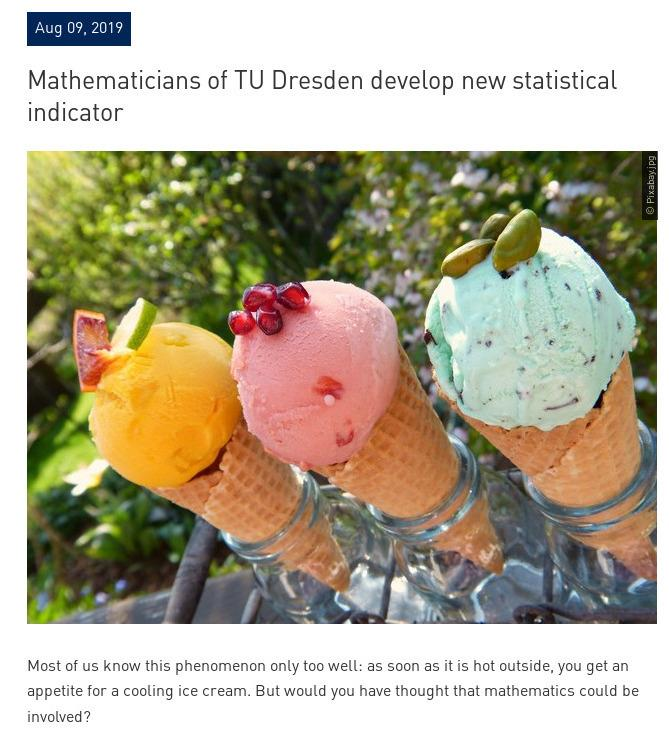# Multivariance

 Science Daily has an article about a new statistical indicator, the ``distance multivariance". It is a non-linear version of the variance. The Science daily article however is just a copy of the TU Dresden public relation branch which sells it with an ice cream story: the consumption of ice cream is correlated with the temperature. Maybe not to use the ice cream analogy also, one can say that the content of the TU news department and the content of the science daily blurb is highly correlated. (This is easy to see because they are identical...), except for the ice cream picture. Click on one of the picture to the right to see the ice cream better.As data are just vectors v and w , the covariance is the dot product v.w (technically, one first centers the vectors by subtracting the mean). The correlation between two vectors is the cosine of the angles between the vectors. It is given by the formula cos(α) = v.w.(|v| |w|) which statisticians write as Cov[v,w]/(σ(v) σ(w)) because the length of the vector is the standard deviation the square root of the variance. Why would one want to introduce other notions of covariance? One reason is that there is a huge difference between ``uncorrelated" and ``independent". The later is much stronger. What the new multivariance tries to capture are more subtle ``nonlinear" type of correlations.
Now, the details of the distance multivariance are a bit tricky (we have to note that this is cutting edge statistics research) but it essentially is a dot product again, after doing a Fourier transform and changing the type of dot product allowing more tuning. In the simplest case, it deals with two random variables x,y (which you can think a out as vectors). If E[X] denotes the expectation (=average) of a random variable, one can look at the complex valued functions f(t) = E[exp(i x t)] and g(t) = E[exp(i y t) which are called the characteristic functions. Now, given some sort of smoothing quantity like exp(-s^2-t^2) the new distance multivariance is defined as
M(x,y) = ∫∫ |E[(exp(i x t) - f(t)) (exp(i y s)-g(s)) ]|2 exp(-t2 - s2) dt ds
The quantity depends on the choice of the measure and since E[X Y] = E[X] E[Y] for independent random variables X,Y, one can see that the quantity M(x,y) is zero if X and Y are independent. In that respect it behaves like covariance. However, due to the different weighting in the Fourier space, the quantity can give more information and possibly detect also more subtle correlations.
The preprint of the article of Björn Böttcher, Martin Keller-Ressel, René L. Schilling "Distance multivariance: New dependence measures for random vectors" The Annals of Statistics, 2019; 47 (5): 2757 is available on The archive.
Looks complicated? Yes it is. But it shows how in statistics higher dimensional integrals (here double integrals) appear naturally. For now, it is better just to eat some ice cream!